# 一符号表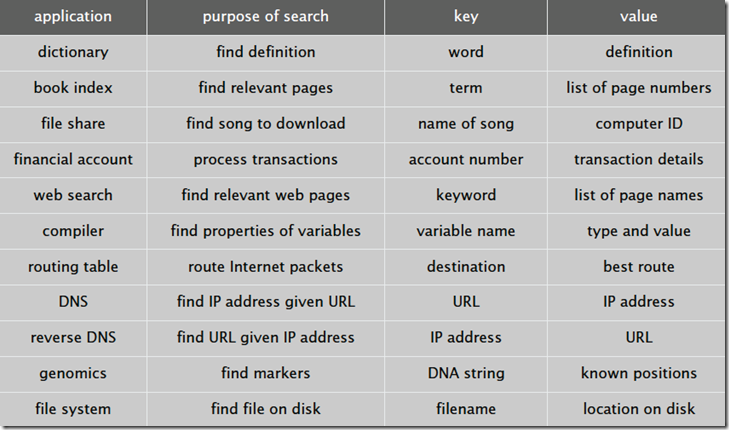public class ST<Key, Value>

 ST() 创建一个查找表对象 void Put(Key key, Value val) 往集合中插入一条键值对记录，如果value为空，不添加 Value Get(Key key) 根据key查找value，如果没找到返回null void Delete(Key key) 删除键为key的记录 boolean Contains(Key key) 判断集合中是否存在键为key的记录 boolean IsEmpty() 判断查找表是否为空 int Size() 返回集合中键值对的个数 Iterable Keys() 返回集合中所有的键

# 二实现

## 1 使用无序链表实现查找表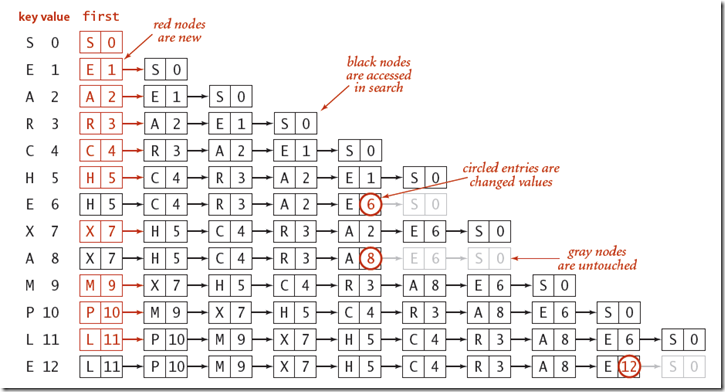public class SequentSearchSymbolTable<TKey, TValue> : SymbolTables<TKey, TValue> where TKey : IComparable<TKey>, IEquatable<TKey>
{
private int length = 0;
Node first;
private class Node
{
public TKey key { get; set; }
public TValue value { get; set; }
public Node next { get; set; }

public Node(TKey key, TValue value, Node next)
{
this.key = key;
this.value = value;
this.next = next;
}
}

public override TValue Get(TKey key)
{
TValue result = default(TValue);
Node temp = first;
while (temp != null)
{
if (temp.key.Equals(key))
{
result = temp.value;
break;
}
temp = temp.next;
}

return result;
}

public override void Put(TKey key, TValue value)
{
Node temp = first;
while (temp != null)
{
if (temp.key.Equals(key))
{
temp.value = value;
return;
}
temp = temp.next;
}
first = new Node(key, value, first);
length++;
}

....
}

## 2 使用二分查找实现查找表

class BinarySearchSymbolTable<TKey, TValue> : SymbolTables<TKey, TValue> where TKey : IComparable<TKey>, IEquatable<TKey>
{
private TKey[] keys;
private TValue[] values;
private int length;
private static readonly int INIT_CAPACITY = 2;
public BinarySearchSymbolTable(int capacity)
{
keys = new TKey[capacity];
values = new TValue[capacity];
length = capacity;
}
public BinarySearchSymbolTable() : this(INIT_CAPACITY)
{
}
/// <summary>
/// 根据key查找value。
/// 首先查找key在keys中所处的位置，如果在length范围内，且存在该位置的值等于key，则返回值
/// 否则，不存在
/// </summary>
/// <param name="key"></param>
/// <returns></returns>
public override TValue Get(TKey key)
{
int i = Rank(key);
if (i < length && keys[i].Equals(key))
return values[i];
else
return default(TValue);
}

/// <summary>
/// 向符号表中插入key，value键值对。
/// 如果存在相等的key，则直接更新value，否则将该key，value插入到合适的位置
///  1.首先将该位置往后的元素都往后移以为
///  2.然后再讲该元素放到为i的位置上
/// </summary>
/// <param name="key"></param>
/// <param name="value"></param>
public override void Put(TKey key, TValue value)
{
int i = Rank(key);
if (i < length && keys[i].Equals(key))
{
values[i] = value;
return;
}
//如果长度相等，则扩容
if (length == keys.Length) Resize(2 * keys.Length);

for (int j = length; j > i; j--)
{
keys[j] = keys[j - 1];
values[j] = values[j - 1];
}

keys[i] = key;
values[i] = value;
length++;
}

/// <summary>
/// 返回key在数组中的位置
/// </summary>
/// <param name="key"></param>
/// <returns></returns>
private int Rank(TKey key)
{
int lo = 0;
int hi = length - 1;
while (lo <= hi)
{
int mid = lo + (hi - lo) / 2;
if (key.CompareTo(keys[mid]) > 0) lo = mid + 1;
else if (key.CompareTo(keys[mid]) < 0) hi = mid - 1;
else return mid;
}
return lo;
}
。。。
}

private int Rank(TKey key, int lo, int hi)
{
if (lo >= hi) return lo;

int mid = lo + (hi - lo) / 2;
if (key.CompareTo(keys[mid]) > 0)
return Rank(key, mid + 1, hi);
else if (key.CompareTo(keys[mid]) < 0)
return Rank(key, lo, hi - 1);
else
return mid;
}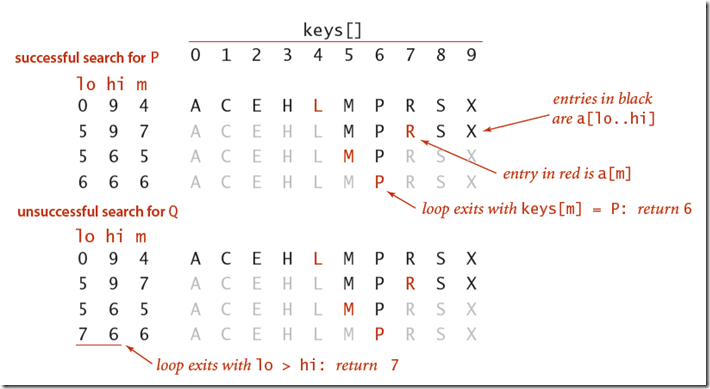# 三 总结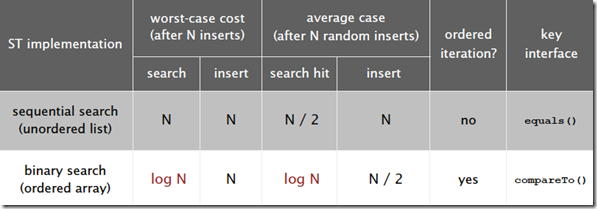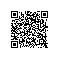使用钉钉扫一扫加入圈子
+ 订阅